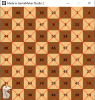# The Knight's Tour

#### Bentley

##### Member
GM Version: GM2

Summary:
The Knight's Tour is a Chess problem where you try to move the knight to every square without revisiting a square. The Warnsdorf algorithm makes this complex problem simple. In this tutorial we will code an animated version of the algorithm. Click the link above to see it run.

A finished Knight's TourAlgorithm
1. Mark the knight's square visited.
2. Look at all the valid squares the knight can move to. A valid square is on the board and unvisited. Give each of those squares a temporary score equal to the number of valid moves a knight could make if it were on that square.
3. Move the knight to the square with the smallest score (ties are ok, just pick one).
4. Repeat 1-3.

Tutorial:
Create Event
GML:
``````#macro COLS 8
#macro ROWS 8
#macro CELL_SIZE (room_width div COLS)
#macro c_light make_color_rgb(241, 194, 125)
#macro c_dark  make_color_rgb(141, 85, 36)

// move_x/y are synced arrays storing the 8 moves the knight can make
move_x = [1, 2, 2, 1, -1, -2, -2, -1];
move_y = [-2, -1, 1, 2, 2, 1, -1, -2];

// This 2D array is initialized to 0 and has two purposes:
// Boolean checks for unvisited squares and for numbering the knight's path through the board (from 1 to 64).
for (var i = 0; i < COLS; i++)
{
for (var j = 0; j < ROWS; j++)
{
visited[i, j] = 0;
}
}

// Save the knight's position
knight_x = irandom(COLS - 1);
knight_y = irandom(ROWS - 1);

// Make the square the knight starts on a part of the tour by setting it to 1
visited[knight_x, knight_y] = 1;

Step Event
GML:
``````// Run the algorithm each step until 64 moves are made
{
// Find the square with the least score
var least = infinity;
var least_x = -1;
var least_y = -1;

// Loop through the moves
for (var i = 0; i < 8; i++)
{
var check_x = knight_x + move_x[i];
var check_y = knight_y + move_y[i];

// A square is valid if it's on the board and unvisited
if (is_valid(check_x, check_y))
{
// Get the square's score and if it's the smallest...
var _score = score_from_square(check_x, check_y);
if (_score < least)
{
// Save the square's (x,y) for moving the knight
// Save the square's score for further comparisions
least_x = check_x;
least_y = check_y;
least = _score;
}
}
}

// Move!
knight_x = least_x;
knight_y = least_y;

// Make this square a part of the tour by setting it to the move number
}``````
score_from_square(x, y)
GML:
``````/// @func score_from_square(x, y)
/// @arg x
/// @arg y
/// @desc Return a score equal to the amount of valid moves from (x,y)

var _x = argument0;
var _y = argument1;
var _score = 0;

// Loop through the moves
for (var i = 0; i < 8; i++)
{
var check_x = _x + move_x[i];
var check_y = _y + move_y[i];

// If the square we're checking is valid, increment the score
if (is_valid(check_x, check_y))
{
_score++;
}
}

return _score;``````
is_valid(x, y)
GML:
``return (inside(argument0, argument1) && !visited[argument0, argument1]);``
inside(x, y)
GML:
``return (point_in_rectangle(argument0, argument1, 0, 0, COLS - 1, ROWS - 1));``
Draw Event
GML:
``````draw_board();

draw_set_halign(fa_center);
draw_set_valign(fa_middle);
draw_set_color(c_black);

// Draw the move number on visited squares
for (var i = 0; i < COLS; i++)
{
for (var j = 0; j < ROWS; j++)
{
if (visited[i, j])
{
var _x = i * CELL_SIZE;
var _y = j * CELL_SIZE;
draw_text(_x + CELL_SIZE / 2, _y + CELL_SIZE / 2, visited[i, j]);
}
}
}

// Draw the knight
draw_sprite_ext(s_knight, 0, knight_x * CELL_SIZE + CELL_SIZE / 2, knight_y * CELL_SIZE + CELL_SIZE / 2, 0.75, 0.75, 0, c_white, 0.66);``````
draw_board()
GML:
``````for (var i = 0; i < COLS; i++)
{
for (var j = 0; j < ROWS; j++)
{
// Alternate light and dark
var color = ((i mod 2 == 0 && j mod 2 == 0) || (i mod 2 != 0 && j mod 2 != 0) ? c_light : c_dark);

// Draw the square
draw_set_color(color);
draw_rectangle(i * CELL_SIZE, j * CELL_SIZE, (i + 1) * CELL_SIZE, (j + 1) * CELL_SIZE, false);
draw_set_color(-1);
}
}``````

Last edited: